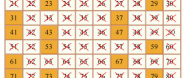## Number.toEratosthenes v1.0A prime number is a natural number greater than 1 that can be divided without remainder only by itself and by 1. Natural numbers n that can be divided by a number less than n and greater than 1 are composite numbers. Sieve of Eratosthenes identifies all prime numbers up to a given number n. The algorithm works as follows: write the numbers 1, 2, 3,4 ,..., n and will eliminate composites by marking them (initially all numbers are unmarked). This is exactly what the method does using bitwise operations.

### Details

Author
Current version
v1.0
GitHub
thinkphp/sieve-of-eratosthenes
3248
Category
Native
Tags
Report
GitHub Issues

• core/1.3: *

### How to use

First you must to include the JS files in the head of your HTML document.

```<script type="text/javascript" src="mootools.js"></script>
<script type="text/javascript" src="number.toEratosthenes.js"></script>
```

```
(function(\$){
if(e) {e.stop();}
var n = \$('n').get('value').toInt();
if(!n) {return false;}
var vec = [];
vec = (n).toEratosthenes();
if(window.console){console.log(vec);}
var s = '';
for(var i=0;i<vec.length;i++) {
s += vec[i] + ', ';
}
});
\$('f').fireEvent('submit');
});
})(document.id);
```

```<form id="f" name="f">
<label for="n">N = </label><input type="text" id="n" value="100"/><input type="submit" value="go">
</form>